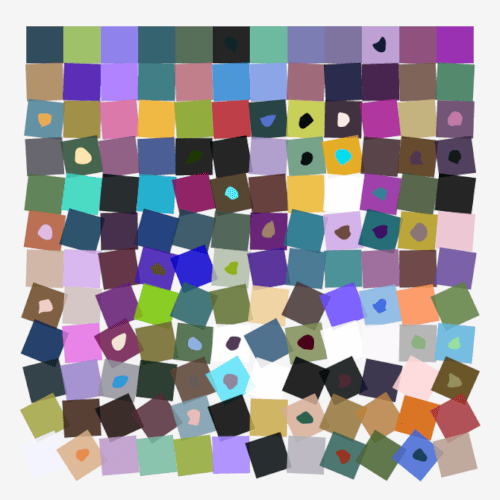## wassily

wassily is both an API and set of tools for creating generative 2D art

### 1 unstable release

 0.1.0 Aug 23, 2023

#375 in Images

MIT/Apache

4.5MB
4.5K SLoC

# Wassily

Wassily is both an API and set of tools for creating generative 2D art. It allows you to create images that can easily be scaled to any size without loss of quality. It is useful for images that are meant to be printed at large sizes or displayed on screen. Included are many generative art algorithms and utilities for dealing with colors and noise.

## Example### `lib.rs`:

Wassily is both an API and set of tools for creating generative 2D art. It allows you to create images that can easily be scaled to any size without loss of quality. It is useful for images that are meant to be printed at large sizes or displayed on screen. Included are many generative art algorithms and utilities for dealing with colors and noise.

## Example``````// Title: Schotter. Inspired by Georg Nees.
use wassily::prelude::*;

const ROWS: u32 = 12;
const COLS: u32 = 12;
const SQUARESIZE: u32 = 50;
const MARGIN: u32 = 35;
const WIDTH: u32 = COLS * SQUARESIZE + 2 * MARGIN;
const HEIGHT: u32 = ROWS * SQUARESIZE + 2 * MARGIN;

fn main() {
// Create a canvas to draw on.
let mut canvas = Canvas::new(WIDTH, HEIGHT);

// We will need a random number generator. 'SmallRng' is reexported from
// the 'rand' crate.
let mut rng = SmallRng::from_entropy();

// Set the background color.
canvas.fill(*WHITESMOKE);

for y in 0..ROWS {
for x in 0..COLS {
let size = SQUARESIZE as f32;
let half_size = size / 2.0;

// Use factor to increase random displacement and angle as we move
// down the rows.
let factor = y as f32 / ROWS as f32;

// Randomly displace the square.
let x_offset = factor * rng.gen_range(-0.5..0.5);
let y_offset = factor * rng.gen_range(-0.5..0.5);

// Calculate the position of the center of the square.
let pos_x = (x * SQUARESIZE + MARGIN) as f32 + x_offset + half_size;
let pos_y = (y * SQUARESIZE + MARGIN) as f32 + y_offset + half_size;

// A random angle to rotate the square.
let angle = factor * rng.gen_range(-45.0..45.0);

// Create a rotation transform.
let rotation = Transform::from_rotate_at(angle, pos_x as f32, pos_y as f32);

// Choose a random color using Okhsl color space, and set it's opacity to 0.75.
let mut fill = rand_okhsl(&mut rng);
fill.set_alpha(0.85);

// Draw the rectangle.
Shape::new()
.rect_cwh(pt(pos_x, pos_y), pt(size, size))
.fill_color(fill)
.no_stroke()
.transform(&rotation)
.draw(&mut canvas);

// Draw a random pearl shape in the middle of the square 25% of the time.
if rng.gen_bool(0.25) {
Shape::new()
// pearl(center, width, height, sides, chaiken iterations, rng)
.pearl(pt(pos_x, pos_y), size / 5.0, size / 5.0, 8, 4, &mut rng)
.fill_color(rand_okhsl(&mut rng))
.no_stroke()
.draw(&mut canvas);
}
}
}
canvas.save_png("./schotter.png");
}
``````

~15MB
~162K SLoC